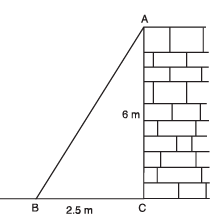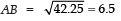Value-Based Questions: Introduction to Trigonometry

# Value-Based Questions: Introduction to Trigonometry - Notes | Study Class 10 Mathematics by VP Classes - Class 10

 1 Crore+ students have signed up on EduRev. Have you?

Q1. A group of students plan to put up a banner in favour of respect towards girls and women, against a wall. They placed a ladder against the wall such that its foot is at a distance of 2.5 m from the wall and its top reaches a window 6 m above the ground.

(i) Find the length of the ladder.
(ii) Which mathematical concept is used in this problem?
(iii) By putting up a banner in favour of respect to women, which value is depicted by the group of students?Sol. (i) Let AB be the ladder and CA be the wall with the window at A. Also,
BC = 2.5 m
CA = 6m
∴ In rt ΔACB, we have
AB2 = BC2 + CA2 [Using Pythagoras Theorem]
= (2.5)2 + (6)2
= 6.25 + 36 = 42.25

⇒Thus, the length of the ladder is 6.5 m.
(ii) Triangles (Pythagoras Theorem)
(iii) Creating positive awareness in public towards regards to women.

The document Value-Based Questions: Introduction to Trigonometry - Notes | Study Class 10 Mathematics by VP Classes - Class 10 is a part of the Class 10 Course Class 10 Mathematics by VP Classes.
All you need of Class 10 at this link: Class 10

## Class 10 Mathematics by VP Classes

132 docs
 Use Code STAYHOME200 and get INR 200 additional OFF

## Class 10 Mathematics by VP Classes

132 docs

Track your progress, build streaks, highlight & save important lessons and more!

,

,

,

,

,

,

,

,

,

,

,

,

,

,

,

,

,

,

,

,

,

;